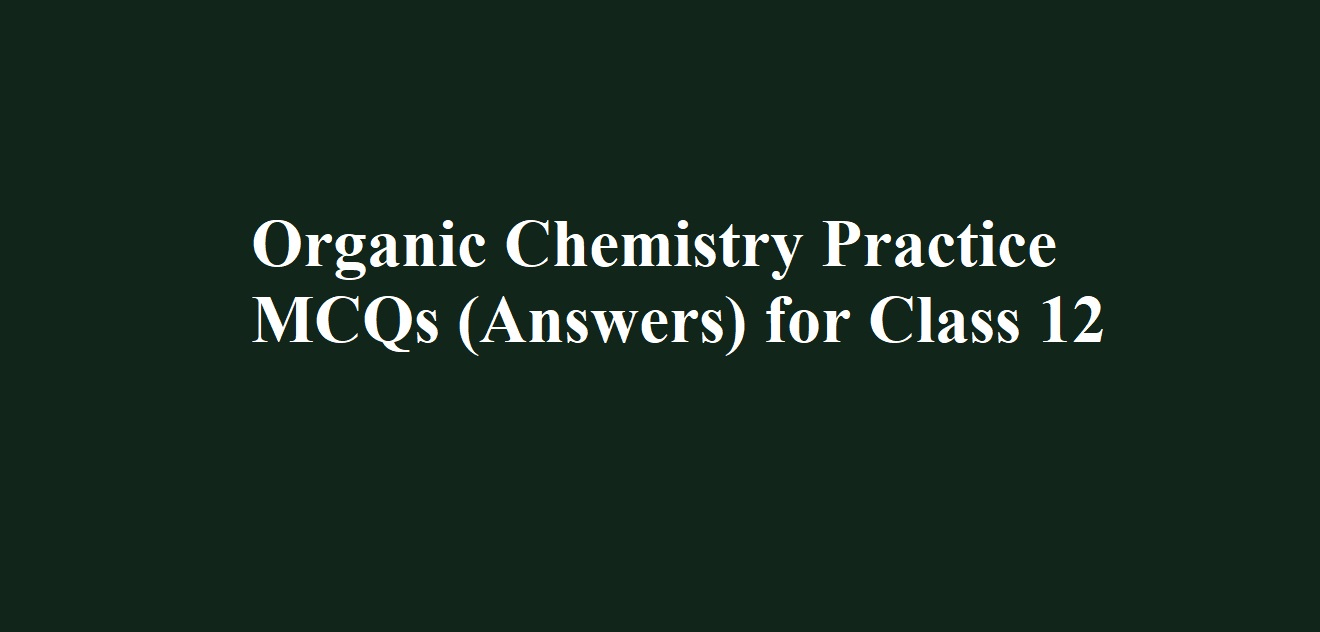# Organic Chemistry Practice MCQs (Answers) for Class 12# Organic Chemistry Practice MCQs (Answers) for Class 12

Organic Chemistry Practice MCQs (Answers) for Class 12

Ans 1. (b) Propan-2-ol

Ans 2. (d) Propanone

Ans 3. (b) 1-Methylcyclohexan-1-ol

Ans 4. (d) X on reaction with Na, does not produce H2 gas

Ans 5. (d) Benzyl alcohol

Ans 6. (c) Treatment with pyridinium chlorochromate

Ans 7. (b) Substitution reaction

Ans 8. (c) B, C

Ans 9. (b)

Ans 10. (b) o-nitrophenol

Ans 11. (d) m-Chlorophenol

Ans 12. (a) Propan-1-ol, butan-2-ol, butan-1-ol, pentan-1-ol

Ans 13. (a) CH3CHOHCH3

Ans 14. (c) Both types

Ans 15. (a) Pri > Sec > Ter
Since Na metal is basic and alcohols are acidic in nature, therefore, the reactivity of Na metal towards alcohols decreases as the acidic strength of alcohols decreases, i.e. in the order 10 > 20 > 30.

Ans 16. (c) The assertion is a correct statement but the reason is the wrong statement.

Ans 17. (d) The assertion is a wrong statement but the reason is the correct statement.

Ans 18. (a) Assertion and reason both are correct and the reason is the correct explanation of assertion.

Ans 19. (c) The assertion is a correct statement but the reason is the wrong statement.

Ans 20. (e) Both assertion and reason are correct statements but the reason is not the correct explanation of assertion.

Ans 21. (a) Assertion and reason both are correct and the reason is the correct explanation of assertion.

Ans 22. (e) Both assertion and reason are correct statements but the reason is not the correct explanation of assertion.

Ans 23. (a) Assertion and reason both are correct and the reason is the correct explanation of assertion.

Ans 24. (d) Replication

Ans 25. (a) An enzyme

Ans 26. (b) A globular protein
Hemoglobin is a globular protein of four subunits, each subunit having a heme moiety and a polypeptide chain (Two α and two β chains).

Ans 27. (a) Does not undergo hydrolysis

Ans 28. (a) Cane sugar

Ans 29. (c) D-glucose

Ans 30. (b) Anomers

Ans 31. (d) Glycine

Ans 32. (b) Monosaccharide

Ans 33. (d) Aldose

Ans 34. (c) H-bond

Ans 35. (d) Both (a) and (b)

Ans 36. (d) Hydroxylamine

Ans 37. (a) n-hexane

Ans 38. (a) Alpha amino acids

Ans 39. (c) 2,4 – DNP reagent

Ans 40. (a) Five

Organic Chemistry Practice MCQs (Answers) for Class 12

To get the Questions Click the Post

Organic Chemistry Practice MCQs for Class 12